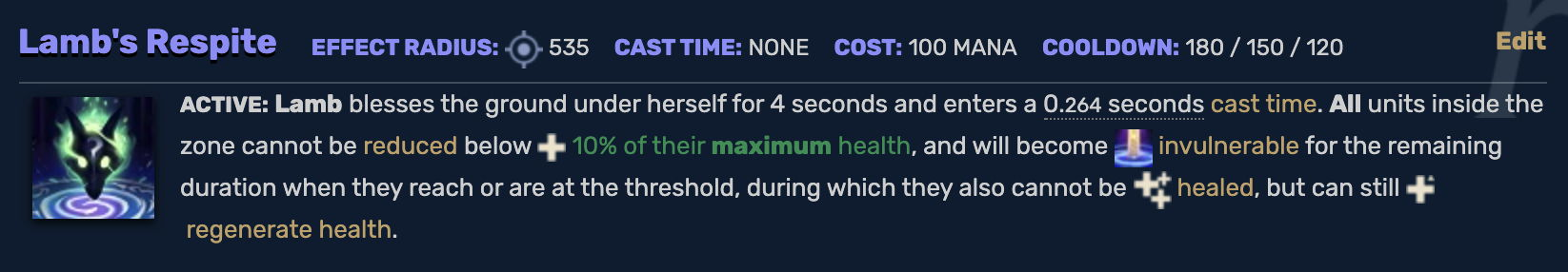시간 제한메모리 제한제출정답맞은 사람정답 비율
3 초 (추가 시간 없음) 1024 MB30111136.667%

## 문제

Wookje is playing the game League of Legends, where two teams of players fight against each other. Each player manages a champion whose status can be represented by two integers: the health $h$, and the maximum health $H$. If a champion's health is less than or equal to zero ($h \le 0$), the champion dies and leaves the game immediately with $h = 0$. If a champion's health is above the maximum health ($h > H$), then it is adjusted to its maximum health. $H$ will always be a positive integer.

In a teamfight, the health of the champion may increase or decrease. More specifically, during a teamfight, the champion was subject to $n$ actions. The $i$-th ($1 \le i \le n$) action increases the health of the champion by $a_i$, subject to the rules above. If $a_i$ is positive, it means the champion was healed. If $a_i$ is negative, it means the champion was attacked. If $a_i$ is zero, then nothing happened to the champion.

Wookje's favorite champion in League of Legends is a lamb named Kindred. Kindred has a ultimate ability named Lamb's Respite. Let's see what this ability does.Formally, consider a champion with maximum health $H$. If Wookje activates Lamb's Respite from right before the $l$-th action until right after the $r$-th action, a champion's health will never fall below $\lceil \frac{H}{10} \rceil$ during these actions. If a champion's health was less than or equal to $\lceil \frac{H}{10} \rceil$ right before the $l$-th action, or would hypothetically be less than or equal to $\lceil \frac{H}{10} \rceil$ after the $i$-th action for some $l \le i \le r$, then its health is set to $\lceil \frac{H}{10} \rceil$ and does not change any further until after the $r$-th action completes. Otherwise, Lamb's Respite does not affect how the champion's health changes.

It is very important to make the right decision on when to activate Lamb's Respite. Wookje wants to improve his decision-making skills. However, the teamfights are too complicated, therefore it's hard for him to know when to activate Lamb's Respite. To help him, please process the following $q$ queries:

• 1 l r x: The champion's maximum health is $x$, and its health starts at $x$. Lamb's Respite is active from right before the $l$-th action to right after the $r$-th action. Output the health of the champion after the $n$ actions. If the champion dies, output $0$. $(1 \le l \le r \le n, 1 \le x \le 10^9)$.
• 2 i x: Update $a_i$ to $x$. $(1 \le i \le n, -10^9 \le x \le 10^9)$.

## 입력

The first line contains two integers, $n$ and $q\ (1 \le n, q \le 300\,000)$.

The second line contains $n$ integers. The $i$-th integer is $a_i\ (|a_i| \le 10^9)$.

The next $q$ lines contain several integers denoting the queries in the described form.

There is at least $1$ query of type $1$.

## 출력

For each query of type $1$, output a single integer denoting the answer to that query. Each answer should go on its own line.

## 예제 입력 1

4 10
0 1 1 -1
1 2 4 2
2 2 -1
1 2 4 2
1 2 3 2
2 1 -1
1 1 4 2
1 2 4 2
2 1 -2
1 1 4 2
1 2 4 2


## 예제 출력 1

1
1
0
1
1
1
0


## 예제 입력 2

6 6
2 5 -3 8 1 -4
1 2 5 7
1 1 3 2
2 2 -1
1 4 6 2
2 1 -1
1 1 6 6


## 예제 출력 2

3
0
0
1